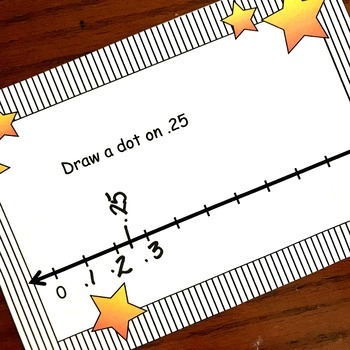# 40 Decimal Task Cards to Introduce Decimals3rd - 5th
Subjects
Standards
Resource Type
Formats Included
• PDF
Pages
20 pages

### Description

Look at an area model or decimal grid and figure out what decimal is represented.
Color in an area model or decimal grid to represent a decimal.
Look at a number line and figure out the decimal represented.
Place a dot on a number line to represent a decimal
Total Pages
20 pages
N/A
Teaching Duration
N/A
Report this Resource to TpT
Reported resources will be reviewed by our team. Report this resource to let us know if this resource violates TpT’s content guidelines.

### Standards

to see state-specific standards (only available in the US).
Compare two decimals to hundredths by reasoning about their size. Recognize that comparisons are valid only when the two decimals refer to the same whole. Record the results of comparisons with the symbols >, =, or <, and justify the conclusions, e.g., by using a visual model.
Use decimal notation for fractions with denominators 10 or 100. For example, rewrite 0.62 as 62/100; describe a length as 0.62 meters; locate 0.62 on a number line diagram.
Express a fraction with denominator 10 as an equivalent fraction with denominator 100, and use this technique to add two fractions with respective denominators 10 and 100. For example, express 3/10 as 30/100, and add 3/10 + 4/100 = 34/100.